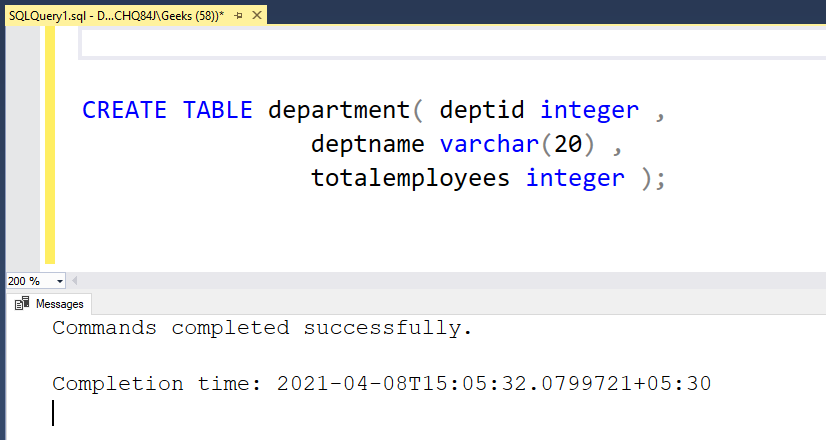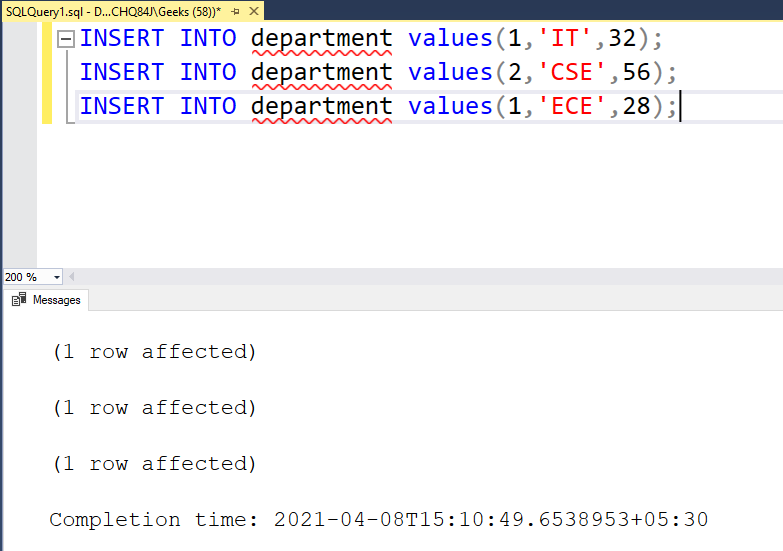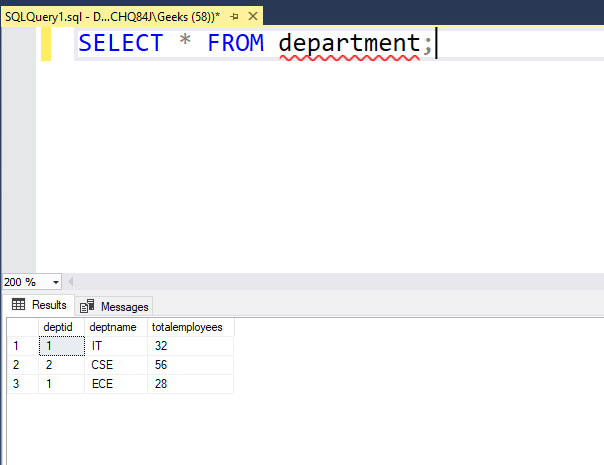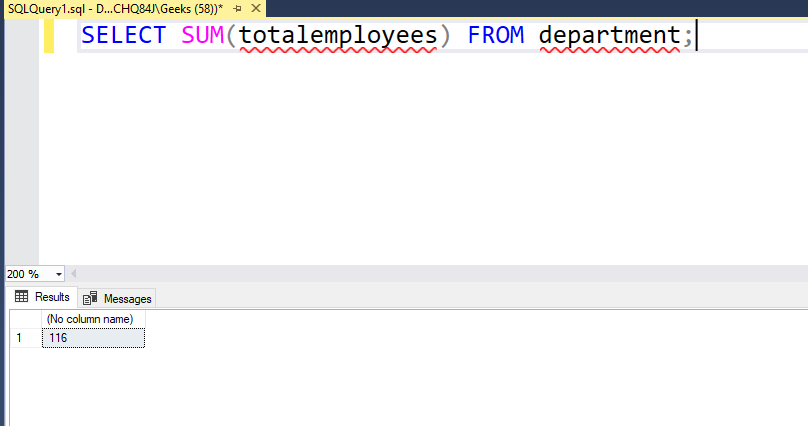Skip to content
Related Articles
SQL Query to Find the Sum of all Values in a Column
• Last Updated : 13 Apr, 2021

In this article, we will look into the process of querying the sum of all values in a column of a database table. But before finding the sum of all values in a column, let us create a table with some columns and data. In this article, we will be using the Microsoft SQL Server as our database.

### Creating a table :

Use the below syntax to create a table inside the database

Syntax :

```create table table_name( column_name 1 data type ( size ) ,
column_name 2 data type ( size) ,
. . . . column_name n data type ( size ) )```

For the sake of illustration, we will be creating a department table and operate on the same. The department table will have 3 fields namely deptid, deptname, totalemployees. To do so use the below statement:

```CREATE TABLE department( deptid integer ,
deptname varchar(20) ,
totalemployees integer );```This will create the table. To insert values into the table we need to use the INSERT statement. So let us see add some data to the department table that we created:

Note: We have to insert values according to the table created. For example, we created a department table with deptid as integer, deptname as varchar, and totalemployees as an integer. So, we need to insert an integer, a character, and an integer respectively.

Now let us insert some rows into the department table using the below query:

```INSERT INTO department values(1,'IT',32);
INSERT INTO department values(2,'CSE',56);
INSERT INTO department values(1,'ECE',28);```

Output:Following the same pattern we have inserted some rows into the table, now let us print the data available in the table using the SELECT statement as shown below:

`SELECT * FROM department;`

Note: Here * represents all. If we execute this query, the entire table will be displayed.

Output :### Sum of all values in a column:

• For this, we need to use the sum() function. We have to pass the column name as a parameter.
• This sum() function can be used with the SELECT query for retrieving data from the table.
• The below example shows to find the sum of all values in a column.

Example :

`SELECT SUM(totalemployees) FROM department;`

Output :Conclusion: Using the sum() function we can get the sum of values in a column using the column name.

Attention reader! Don’t stop learning now. Get hold of all the important CS Theory concepts for SDE interviews with the CS Theory Course at a student-friendly price and become industry ready.

My Personal Notes arrow_drop_up